# 第 1 章 绪论

## 1.1 学科介绍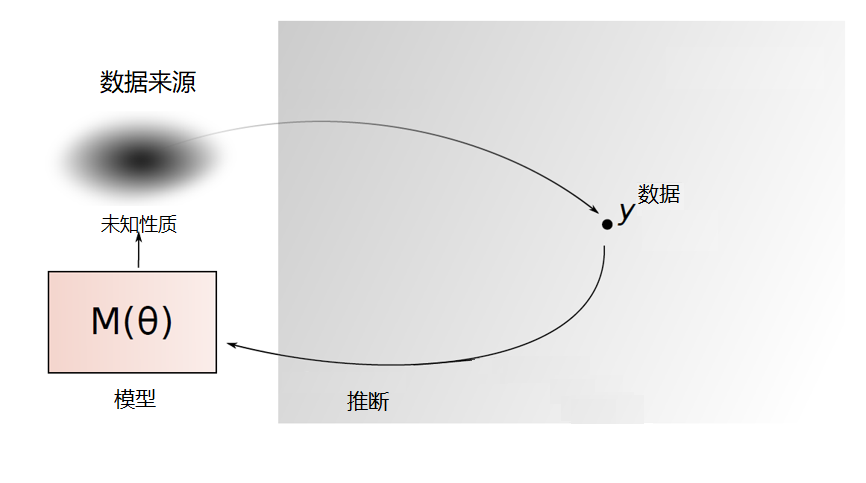Essentially, all models are wrong, but some are useful. —— George Box

1. 参数估计1. 假设检验### 1.1.1 统计学的发展简史

1. 第一个时期（古典统计学时期）

1. 第二个时期（近代统计学时期）

20世纪初至第二次世界大战为近代统计学发展时期。科学技术开始进入全面繁荣时期，天文、气象、社会人口等领域的数据资料达到一定规模的积累，对统计的需求已从国家层面扩展至社会科学各个领域。对事物现象静态性的描述也已不能满足社会需求。一些重要的统计概念也在这一时期提出，误差测定、正态分布曲线、最小二乘法、大数定律等理论方法的大量运用为社会、经济、人口、法律等领域的研究提供了大量宝贵的指导。代表性人物：费希尔(R. A. Fisher, 1890-1962), 奈曼(J. Neyman, 1894-1981), 小皮尔逊(E. S. Pearson, 1895-1980), 许宝騄(1910-1970)等。

1. 第三个时期（现代统计学时期）

20世纪80年代开始，随着现代生物医学的发展，计算机技术的进步，人类对健康的管理和疾病的治疗已进入基因领域，对基因数据分析产生了大量需求。高维海量的基因数据具有全新的数据特征，变量维度远远大于样本数，传统的统计方法失效了，因此一系列面向高维数据的统计分析方法相继产生，比如著名的Lasso方法。

20世纪90年代以来，随着互联网的发展，数据库中积累了海量的数据，进入大数据时代。如何从海量的数据中挖掘有用的信息就变得越来越重要了，数据挖掘也就应运而生了。与数据挖掘比较接近的名词是机器学习, 机器学习被看作是人工智能的一个分支，主要是研究一些让计算机可以自动“学习”的算法，是一类从数据中自动分析获得规律，并利用规律对未知数据进行预测的算法。因为机器学习算法中涉及了很多的统计学理论，与统计学的关系密切，也被称为统计学习

### 1.1.2 频率学派与贝叶斯学派

• 频率学派认为样本信息来自总体，仅通过研究样本信息可以对总体信息做出合理的推断和估计，并且样本越多，就越准确。

• 代表性人物：费希尔 (R. A. Fisher, 1890-1962)

• 起源于英国学者贝叶斯(T. Bayes, 1702-1761)在1763年发表的著名论文《论有关机遇问题的求解》
• 最基本观点：任何一个未知量都可以看作是随机的，应该用一个概率分布去描述未知参数，而不是频率派认为的固定值。这种信息称为先验信息，是主观信息。

Good (1973)评价道：

“主观主义者直抒他们的判断，而客观主义者以假设来掩盖其判断，并以此享受科学客观性的荣耀。”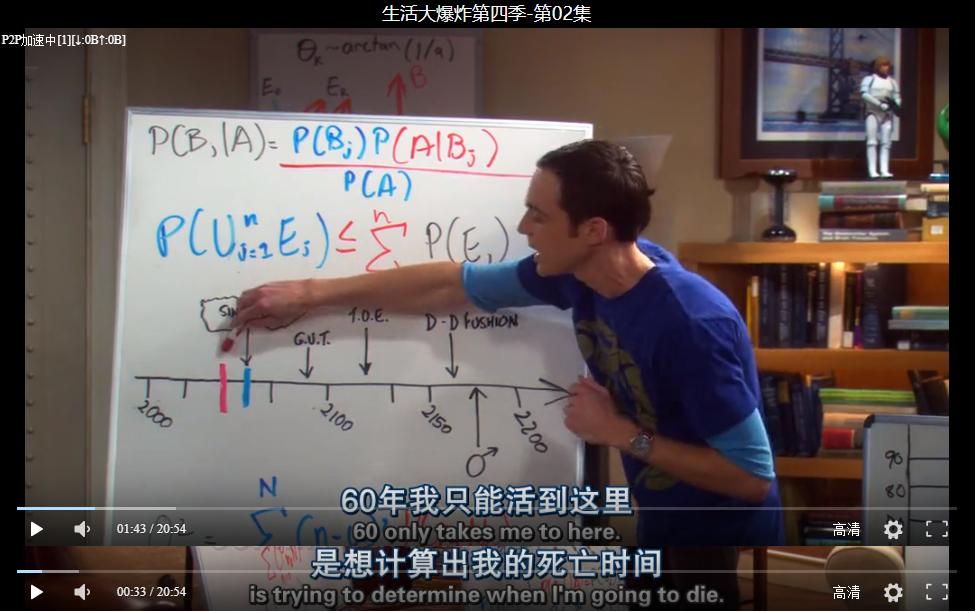• 自然语言处理：计算机翻译语言、识别语音、认识文字和海量文献的检索

• 人工智能、无人驾驶

• 垃圾短信、垃圾邮件识别

• 如何在一个陌生的地方找餐馆吃饭？

### 1.1.3 统计学专业

1. 统计学专业包含理论统计和应用统计两方面
• 理论统计：模型选择，非参统计方法，贝叶斯统计，时间序列与生存分析，高维数据分析与机器学习，数据挖掘等等。

• 应用统计：目前发展最为突出的是生物统计，金融统计等等。

1. 统计学经过漫长的发展，尤其是计算机的大量应用，目前包括但不限于下面这些分支（或者交叉领域）。目前最火热的学科都是跟计算机结合比较紧密的。
• 统计理论研究：大样本性质、各种渐近理论分析等
• 高维（超高维）统计推断：变量选择、大规模假设检验
• 统计计算方法：蒙特卡罗模拟、卡尔曼滤波算法、近似贝叶斯算法、自助法
• 生物统计：纵向分析、空间分析
• 统计学习：数据挖掘、人工智能

## 1.2 基本概念

### 1.2.1 总体

• 网上购物居民占全市居民的比例
• 过去一年内网购居民的购物次数
• 某品牌灯泡的寿命

### 1.2.2 样本

1. 普查（全数检查）
• 对总体中的每个个体进行观察，如我国每十年一次的人口普查
• 缺点：费用高、时间长、不适合破坏性试验
1. 抽样
• 从总体中抽取若干个体进行观察，用所获得数据对总体进行统计推断
• 优点：费用低、时间短
• 抽取的部分组成的集合$$(X_1,\dots,X_n)$$称为样本$$X_i$$称为样品
• 样品个数$$n$$称为样本量或者样本容量

### 1.2.3 简单随机抽样

• 随机性：每个个体都有相同的机会选中（有放回随机抽取/独立重复观测），即$$X_i$$与总体$$X$$同分布
• 独立性：每个样本的选取是独立的

• 抽取之前无法预知它们的数值，故$$(X_1,\dots,X_n)$$$$n$$维随机向量
• 抽取后样本为具体的数，用小写字母$$(x_1,\dots,x_n)$$表示，称为样本观测值

### 1.2.4 案例

2018年高考全国II卷作文（适用地区: 内蒙古、黑龙江、辽宁、吉林、重庆、陕西、甘肃、宁夏、青海、新疆、西藏、海南）

“二战”期间，为了加强对战机的防护，英美军方调查了作战后幸存飞机上弹痕的分布，决定哪里弹痕多就加强哪里，然而统计学家瓦尔德(Abrahom Wald, 1902–1950)力排众议，指出更应该注意弹痕少的部位，因为这些部位受到重创的战机，很难有机会返航，而这部分数据被忽略了。事实证明沃德是正确的。## 1.3 概率分布族

1. 参数族
• $$\mathcal{F}$$中的分布的一般数学形式已知，但包含若干未知参数$$\theta=(\theta_1,\dots,\theta_m)$$
• $$\mathcal{F}:=\{F_\theta,\theta\in\Theta\}$$, 其中$$\Theta\subset \mathbb{R}^m$$称为参数空间
• 该模型为参数统计问题$$m$$为模型的维数
• $$m=1$$为单参数统计问题，$$m>1$$为多参数统计问题
1. 非参数族
• $$\mathcal{F}$$中的分布不能通过有限个未知参数来刻画
• 该模型为非参数统计问题
1. 半参数族
• $$\mathcal{F}$$中的分布有一部分可以用参数刻画，一部分则不可以。

### 1.3.1 常用的参数族

1. 离散型
• 二项分布族$$\{b(n,p);0<p<1\}$$
• 几何分布族$$\{Ge(p);0<p<1\}$$
• 泊松分布族$$\{P(\lambda);\lambda>0\}$$
1. 连续型
• 正态分布族$$\{N(\mu,\sigma^2);-\infty<\mu<\infty,\sigma>0\}$$
• 均匀分布族$$\{U(a,b);-\infty<a<b<\infty\}$$
• 指数分布族$$\{Exp(\lambda);\lambda>0\}$$

### 1.3.2 伽玛分布族

$f(x) = \frac{\lambda^\alpha}{\Gamma(\alpha)}x^{\alpha-1}e^{-\lambda x}1\{x> 0\},$ 其中$$\alpha>0$$称为形状参数，$$\lambda>0$$称为速率，$$1/\lambda$$称为尺度参数，$$\Gamma(\alpha)$$为伽马函数，定义及性质如下：

• $$\Gamma(\alpha)=\int_0^{+\infty} x^{\alpha-1}e^{-x}dx$$
• $$\Gamma(1)=1,\Gamma(1/2)=\sqrt{\pi}$$
• $$\Gamma(\alpha+1)=\alpha\Gamma(\alpha)$$
• $$\alpha$$为整数$$n$$时，$$\Gamma(n+1)=n!$$

• $$\alpha=1$$时伽玛分布为指数分布，即$$Ga(\alpha,\lambda)=Exp(\lambda)$$
• $$\alpha=n/2,\lambda=1/2$$时伽玛分布为自由度为$$n$$的卡方分布（后文提及），即$$Ga(n/2,1/2)=\chi^2(n)$$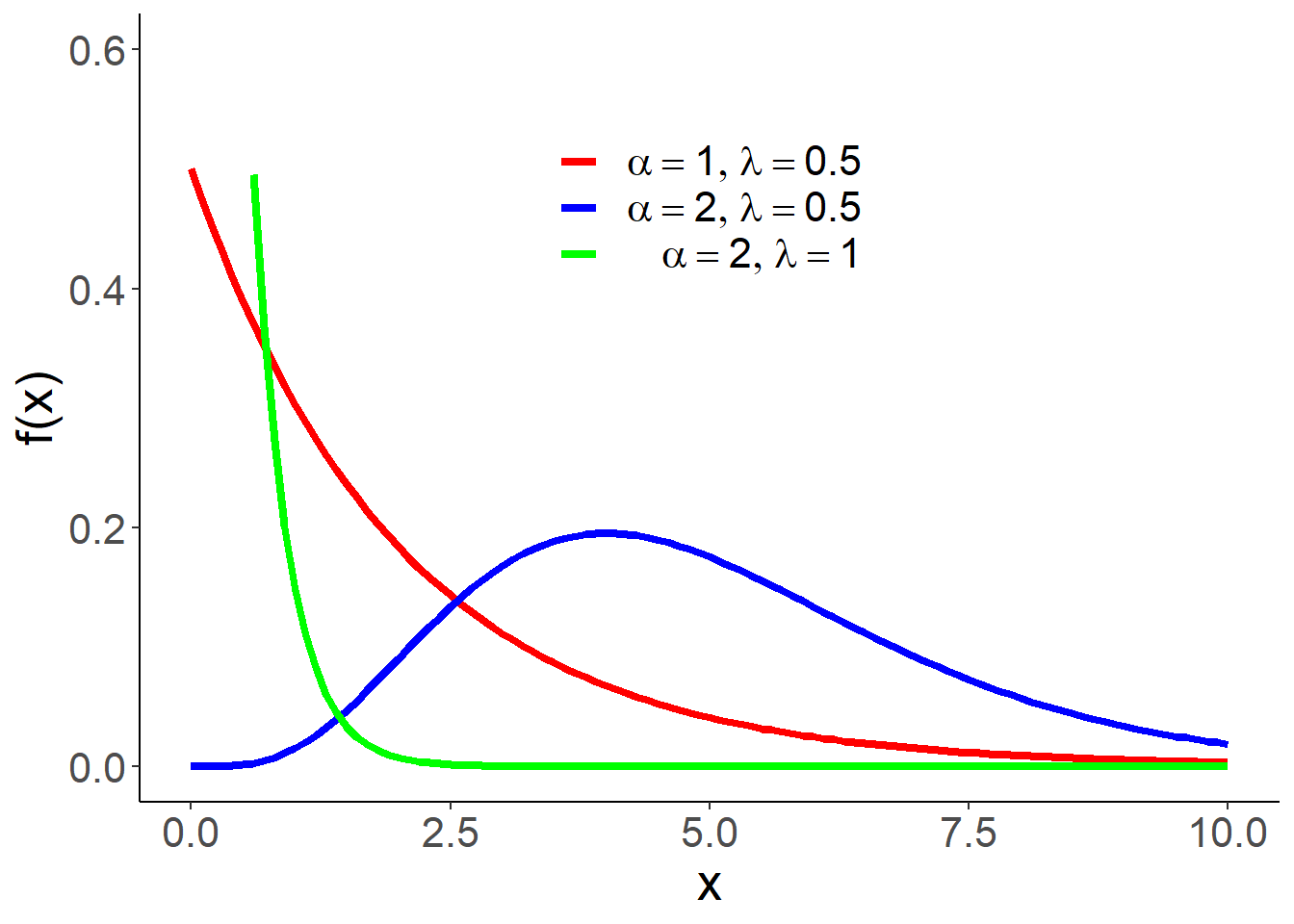$\phi(t)=E[e^{itX}]=\left(1-\frac{it}\lambda\right)^{-\alpha}.$

### 1.3.3 贝塔分布族

$f(x) = \frac{\Gamma(\alpha+\beta)}{\Gamma(\alpha)\Gamma(\beta)}x^{\alpha-1}(1-x)^{\beta-1}1\{0<x<1\},$ 其中$$\alpha>0,\beta>0$$为形状参数族。记为$$Beta(\alpha,\beta)$$，贝塔分布期望为$$\frac{\alpha}{\alpha+\beta}$$，方差：$$\frac{\alpha \beta}{(\alpha+\beta)^2(\alpha+\beta+1)}$$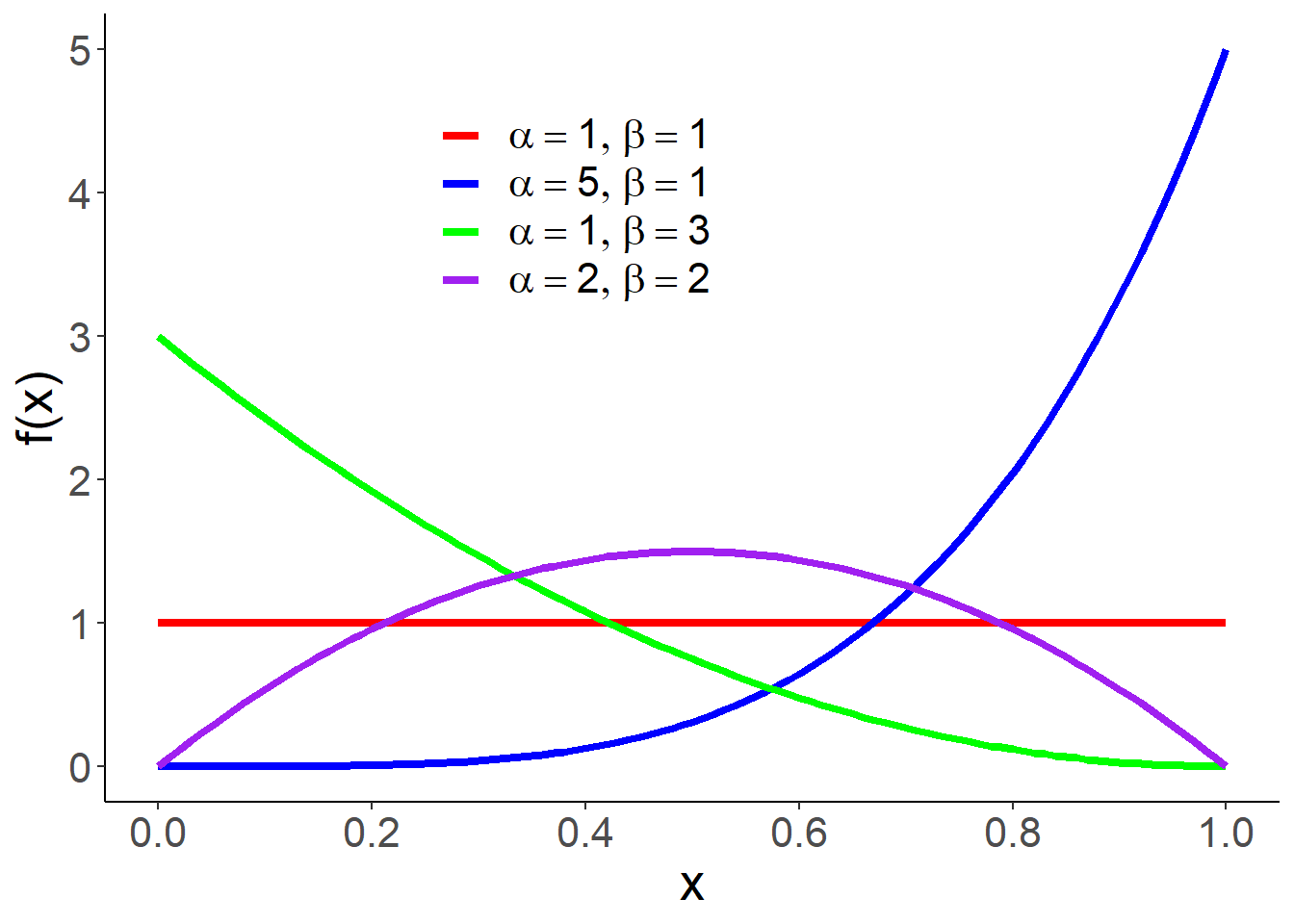• 不合格率
• 市场占有率
• 命中率

### 1.3.4 指数型分布族

$f_\theta(x)=c(\theta)\exp\{\sum_{j=1}^kc_j(\theta)T_j(x)\}h(x),$

• $$k$$为正整数，称为该指数分布族的“维数”。
• $$c(\theta)>0,c_j(\theta)$$为参数空间$$\Theta$$上的函数
• $$h(x)\ge 0$$, $$T_1(x),\dots,T_k(x)$$线性无关

$$\eta_j=c_j(\theta),\eta=(\eta_1,\dots,\eta_k)^\top$$，则分布函数可以变成关于新参数$$\eta$$的函数

$f_\eta(x)=\tilde c(\eta)\exp\{\sum_{j=1}^k\eta_j T_j(x)\}h(x),$ 其中

$\tilde c(\eta) = 1/\int \exp\{\sum_{j=1}^k\eta_j T_j(x)\}h(x)d x.$$$\eta_i$$为自然参数。

1. 正态分布族是指数型分布族

$f(x,\mu,\sigma)=\frac 1{\sqrt{2\pi}\sigma}e^{-\mu^2/(2\sigma^2)}\exp\{\frac{\mu}{\sigma^2}x-\frac{1}{2\sigma^2}x^2\}$

1. 二项分布族是指数型分布族

$P(X=x) = C_n^x p^x(1-p)^{n-x}=(1-p)^n\exp\{\ln[p/(1-p)]x \}C_n^x$

1. 伽玛/贝塔分布族是指数型分布族（请自行验证）

$f_\theta(x_1,\dots,x_n)=\prod_{i=1}^np_\theta(x_i)=c(\theta)^n\exp\{\sum_{j=1}^kc_j(\theta)\sum_{i=1}^nT_j(x_i)\}\prod_{i=1}^nh(x_i).$

## 1.4 统计量与估计量

$T=T(X_1,\dots,X_n):\mathbb{R}^n\to \mathbb{R}$不含有任何未知参数的（可测）函数，则称$$T$$为统计量。统计量的分布称为抽样分布

• 分布中所含的未知参数
• 分布的数字特征：期望、方差、标准差、分位数等
• 某事件的概率

$T_1 = \frac{\sqrt{n}(\sum_{i=1}^n X_i-\mu)}{\sigma},$

$T_2 = \min(X_1,\dots,X_n).$

• 样本均值

$\bar{X}=\bar X_n=\frac{1}{n}\sum_{i=1}^n X_i$

• 样本方差

$S_n^2=\frac{1}{n}\sum_{i=1}^n (X_i-\bar{X})^2$

• 修正样本方差

$S_n^{*2}=\frac{1}{n-1}\sum_{i=1}^n (X_i-\bar{X})^2=\frac{n}{n-1}S_n^2$

• 样本标准差

$S_n=\sqrt{S_n^2}$

• 样本$$k$$阶原点矩 $\overline{X^k}=\frac{1}{n}\sum_{i=1}^n X_i^k$

• 样本$$k$$阶中心矩 $\frac{1}{n}\sum_{i=1}^n (X_i-\bar X)^k$

• 顺序统计量 $X_{(1)}\le X_{(2)}\le \dots\le X_{(n)}$

• 样本中位数

$\tilde{X}= \begin{cases} X_{(\frac{n+1}{2})},\ &\text{n为奇数}\\ (X_{(\frac{n}{2})}+X_{(\frac{n}{2}+1)})/2,\ &\text{n为偶数} \end{cases}$

## 1.5 充分统计量

• 简化数据
• 不损失样本信息

：上面是充分统计量的数学定义，该定义如何体现“不损失样本信息”这一特征呢？假设现在只知道充分统计量$$T$$的观测值为$$t$$，是否可以由此“恢复”样本数据？注意到在给定$$T=t$$条件下，样本$$(X_1,\dots,X_n)$$的条件分布与参数$$\theta$$无关，我们可以通过这个条件分布随机生成样本$$(X'_1,\dots,X'_n)$$。（为什么？）容易证明，$$(X'_1,\dots,X'_n)$$的分布与$$(X_1,\dots,X_n)$$一样。从这个角度上讲，充分统计量不损失样本信息。

• $$T_1=\sum_{i=1}^nX_i$$
• $$T_2=X_1+X_2$$

\begin{align*} P(X_1=x_1,\dots,X_n=x_n|T_1=t) &= \frac{P(X_1=x_1,\dots,X_n=x_n,T_1=t)}{P(T_1=t)}\\ &=\begin{cases} \frac{P(X_1=x_1,\dots,X_n=x_n)}{P(T_1=t)},\ &\sum_{i=1}^n x_i = t\\ 0, & else \end{cases} \\ &=\begin{cases} \frac{p^{\sum_{i=1}^n x_i}(1-p)^{n-\sum_{i=1}^n x_i}}{C_n^t p^t(1-p)^{n-t}},\ &\sum_{i=1}^n x_i = t\\ 0, & else \end{cases} \\&=\begin{cases} \frac 1{C_n^t},\ &\sum_{i=1}^n x_i = t\\ 0, & else \end{cases} \end{align*} 该分布与$$p$$无关，所以$$T_1$$$$p$$的充分统计量。这意味着，如果知道$$T_1=t$$，则$$(X_1,\dots,X_n)$$的取值只有$$C_n^t$$种，每种情况发生的可能性一样。此时，只需要在这$$C_n^t$$种情况中等可能抽样就可以“恢复数据”。

\begin{align*} P(X_1=x_1,\dots,X_n=x_n|T_2=t) &= \frac{P(X_1=x_1,\dots,X_n=x_n,T_2=t)}{P(T_2=t)} \\&=\frac{P(X_1=x_1,X_2=t-x_1,\dots,X_n=x_n)}{P(T_2=t)} \\&=\frac{p^{t+\sum_{i=3}^n x_i}(1-p)^{n-t-\sum_{i=3}^n x_i}}{C_2^t p^t(1-p)^{2-t}} \\&=\frac 1{C_2^t}p^{\sum_{i=3}^n x_i}(1-p)^{n-2-\sum_{i=3}^n x_i} \end{align*}

\begin{align*} P(X_1=x_1,\dots,X_n=x_n|T=t) &=\frac{P(X_1=x_1,\dots,X_n=x_n,T=t)}{P(T=t)} \\&=\frac{\prod_{i=1}^n[p(1-p)^{x_i-1}]}{C_{t-1}^{n-1} p^n (1-p)^{t-n}}=\frac{1}{C_{t-1}^{n-1}} \end{align*} 该分布与$$p$$无关，所以$$T$$$$p$$的充分统计量。

\begin{align*} f_\theta(x_1,\dots,x_n|T(x_{1:n})=t)&=\frac{f_\theta(x_1,\dots,x_n,t)}{f^T_\theta(t)} \\&=\frac{f_\theta(t|x_1,\dots,x_n)f_\theta(x_1,\dots,x_n)}{f^T_\theta(t)} \\&= \frac{\prod_{i=1}^nf_\theta(x_i)1\{T(x_1,\dots,x_n)=t\}}{f^T_\theta(t)}. \end{align*}

### 1.5.1 因子分解定理

1. $$h(x_1,\dots,x_n)$$非负
2. 在统计量$$T$$取值空间上的函数$$g_\theta(t)$$, 使得
$\begin{equation} f_\theta(x_1,\dots,x_n) = g_\theta(T(x_1,\dots,x_n))h(x_1,\dots,x_n),\ \forall\theta\in\Theta. \tag{1.1} \end{equation}$

（1）必要性

$\{X_{1:n}=x_{1:n}\}\subset \{T(X_{1:n})=T(x_{1:n})\}.$

\begin{align*} P(X_{1:n}=x_{1:n})&= P(X_{1:n}=x_{1:n}, T(X_{1:n})=T(x_{1:n})) \\&=P(X_{1:n}=x_{1:n}|T(X_{1:n})=T(x_{1:n}))P(T(X_{1:n})=T(x_{1:n})) \\&=h(T(x_{1:n}),x_{1:n})g_\theta(T(x_{1:n})), \end{align*} 其中$$g_\theta$$$$T$$的分布函数。

（2）充分性 设样本$$X_{1{:}n}$$的取值空间为$$\Omega$$，令$$A(t) = \{x_{1:n}\in \Omega|T(x_{1:n})=t\}$$. 不难看出集合$$A(t)$$有至多可列个元素。 \begin{align*} P(X_{1:n}=x_{1:n}|T(X_{1:n})=t)) &= \frac{P(X_{1:n}=x_{1:n},X_{1:n}\in A(t))}{P(X_{1:n}\in A(t))}\\ &= \frac{P(X_{1:n}=x_{1:n})1\{x_{1:n}\in A(t)\}}{\sum_{y_{1:n}\in A(t)}P(X_{1:n}=y_{1:n})}\\ &= \frac{g_\theta(T(x_{1:n}))h(x_{1:n})1\{x_{1:n}\in A(t)\}}{\sum_{y_{1:n}\in A(t)}g_\theta(T(y_{1:n}))h(y_{1:n})}\\ &= \frac{g_\theta(t)h(x_{1:n})1\{x_{1:n}\in A(t)\}}{\sum_{y_{1:n}\in A(t)}g_\theta(t)h(y_{1:n})}\\ &= \frac{h(x_{1:n})1\{x_{1:n}\in A(t)\}}{\sum_{y_{1:n}\in A(t)}h(y_{1:n})} \end{align*}$$\theta$$无关，所以是充分的。

### 1.5.2 因子分解定理的应用

$f_\theta(x_1,\dots,x_n) =\prod_{i=1}^n \frac 1\theta 1\{0<x_i<\theta\}=\frac 1{\theta^n}1\{x_{(1)}>0\}1\{x_{(n)}<\theta\}.$

• 参数$$(\mu,\sigma^2)$$的充分统计量
• $$\sigma^2$$已知时，$$\mu$$的充分统计量
• $$\mu$$已知时，$$\sigma^2$$的充分统计量

$f_\theta(x_1,\dots,x_n) = \frac{1}{(2\pi)^{n/2}}e^{-\frac{\sum_{i=1}^n(x_i-\mu)^2}{2\sigma^2}}=\frac{1}{(2\pi)^{n/2}}e^{-\frac{\sum_{i=1}^n x_i^2-2\mu\sum_{i=1}^n x_i +n\mu^2}{2\sigma^2}}.$

$f_\theta(x_1,\dots,x_n)=\prod_{i=1}^np_\theta(x_i)=c(\theta)^n\exp\{\sum_{j=1}^kc_j(\theta)\sum_{i=1}^nT_j(x_i)\}\prod_{i=1}^nh(x_i)$

$\left(\sum_{i=1}^nT_1(x_i),\dots,\sum_{i=1}^nT_k(x_i)\right).$

• $$(\bar X, S_n)$$
• $$(\bar X, S_n^2)$$
• $$(\bar X, S_n^*)$$
• $$(\bar X, S_n^{*2})$$
• $$(\sum_{i=1}^n X_i,\sum_{i=1}^n X_i^2)$$
• $$(\sum_{i=1}^n X_i,\sum_{i=1}^n |X_i|)$$
• $$(\sum_{i=1}^n X_i,\sum_{i=1}^n |X_i|,\sum_{i=1}^n X_i^2)$$

## 1.6 抽样分布

1. 精确抽样分布
• 当总体$$X$$的分布已知时，对任意自然数$$n$$都能导出统计量$$T(X_1,\dots,X_n)$$的分布的显示表达式
• 对样本量$$n$$较小的统计推断问题（小样本问题）特别有用
• 精确抽样分布多数是在正态总体下得到
1. 渐近抽样分布
• 寻求在样本量$$n$$无限大时统计量$$T(X_1,\dots,X_n)$$的极限分布
• 适用于对样本量$$n$$较大的统计推断问题（大样本问题）
• 常用的方法是中心极限定理
1. 近似抽样分布
• 寻找一种分布来近似统计量$$T(X_1,\dots,X_n)$$的分布

### 1.6.1 样本均值的抽样分布

• 如果$$X\sim N(\mu,\sigma^2)$$，则$$\bar X$$的精确分布为$$N(\mu,\sigma^2/n)$$.
• 如果总体不是正态分布，但$$E[X]=\mu,Var[X]=\sigma^2$$存在，则$$\bar X$$的渐近分布为 $$N(\mu,\sigma^2/n)$$，记为$$\bar X\stackrel{\cdot}\sim N(\mu,\sigma^2/n)$$.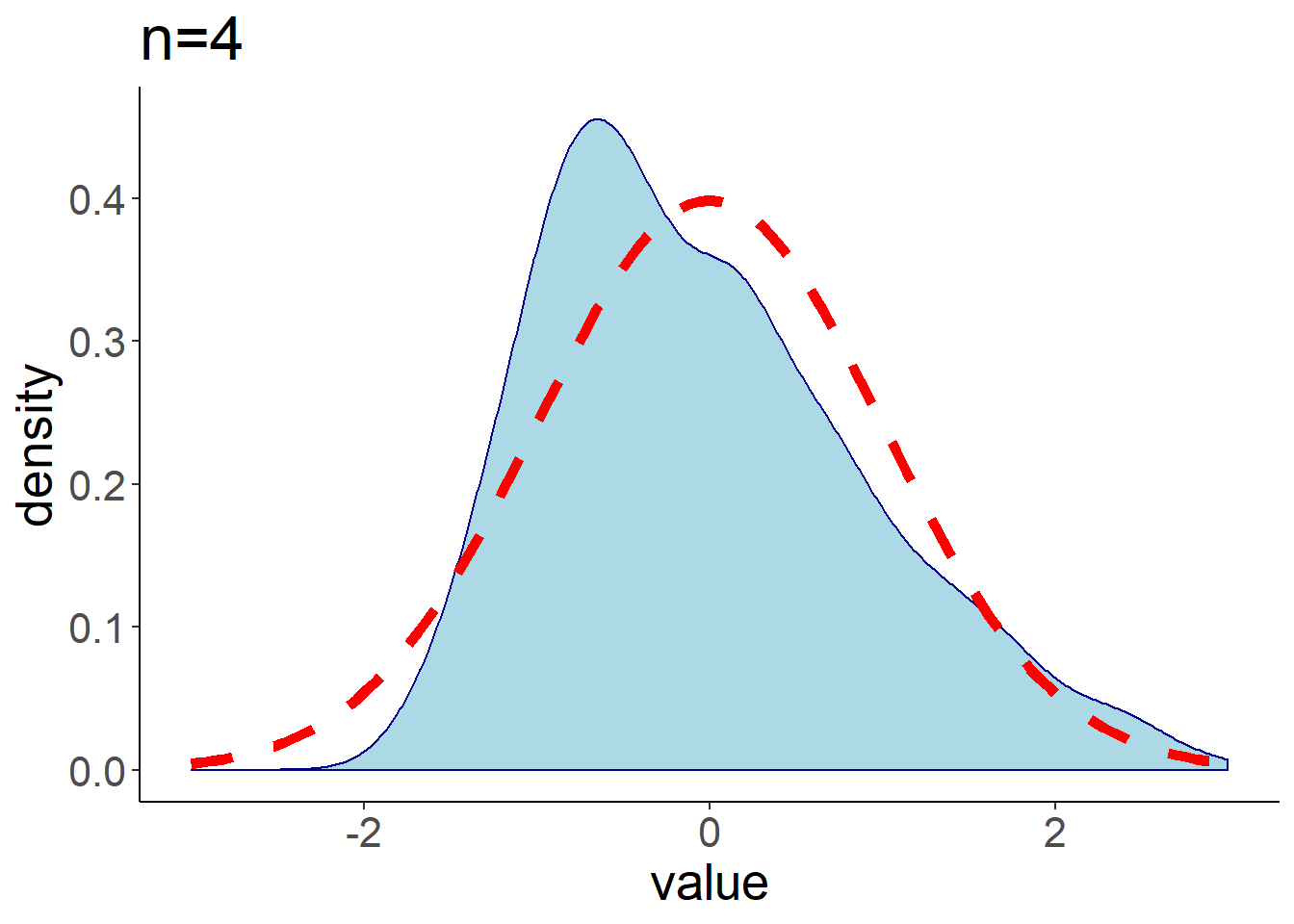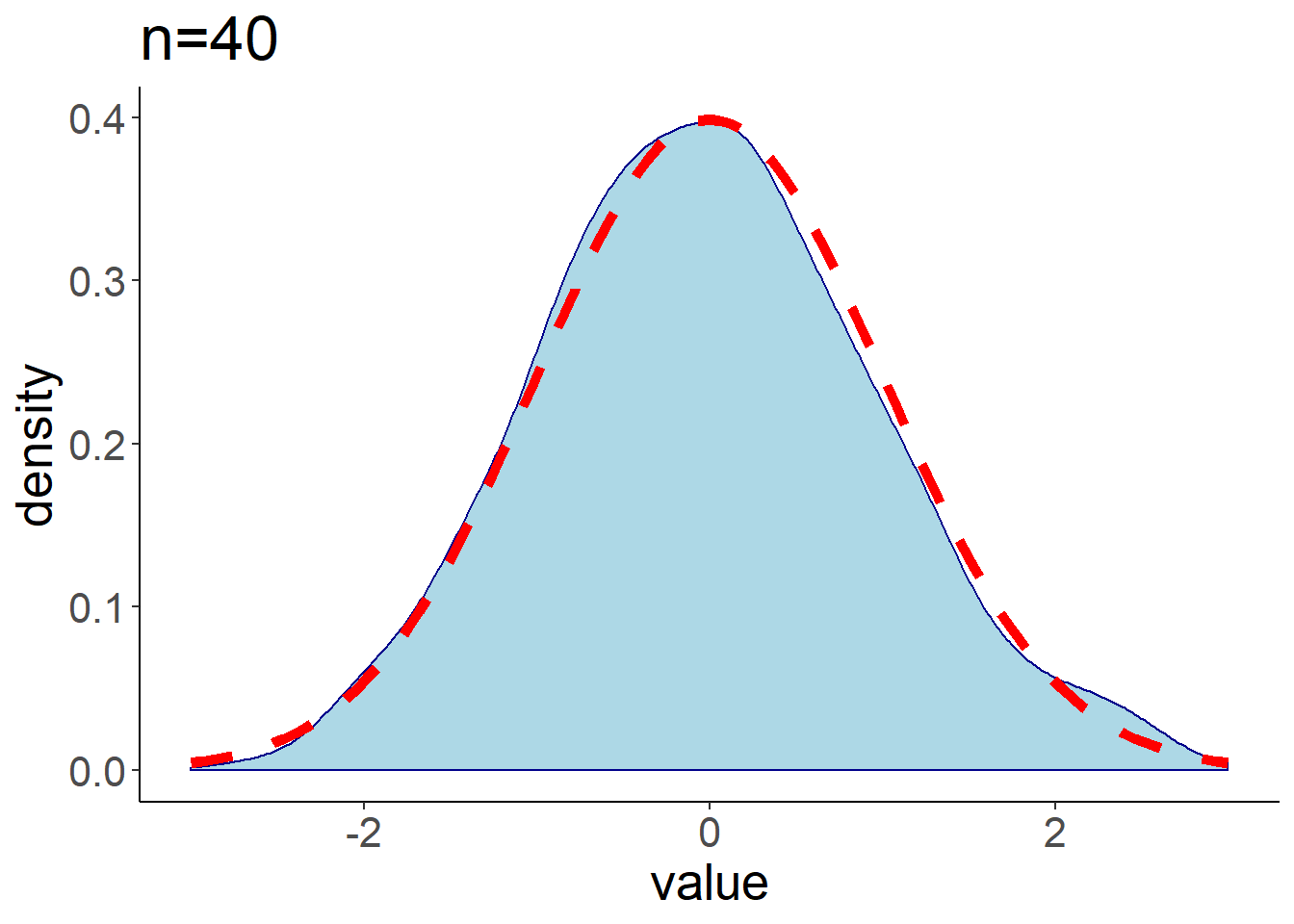### 1.6.2 卡方分布

$f_n(x)=\frac{1}{2^{\frac n2}\Gamma(n/2)}x^{\frac n2-1}e^{-\frac x2} 1\{x>0\}.$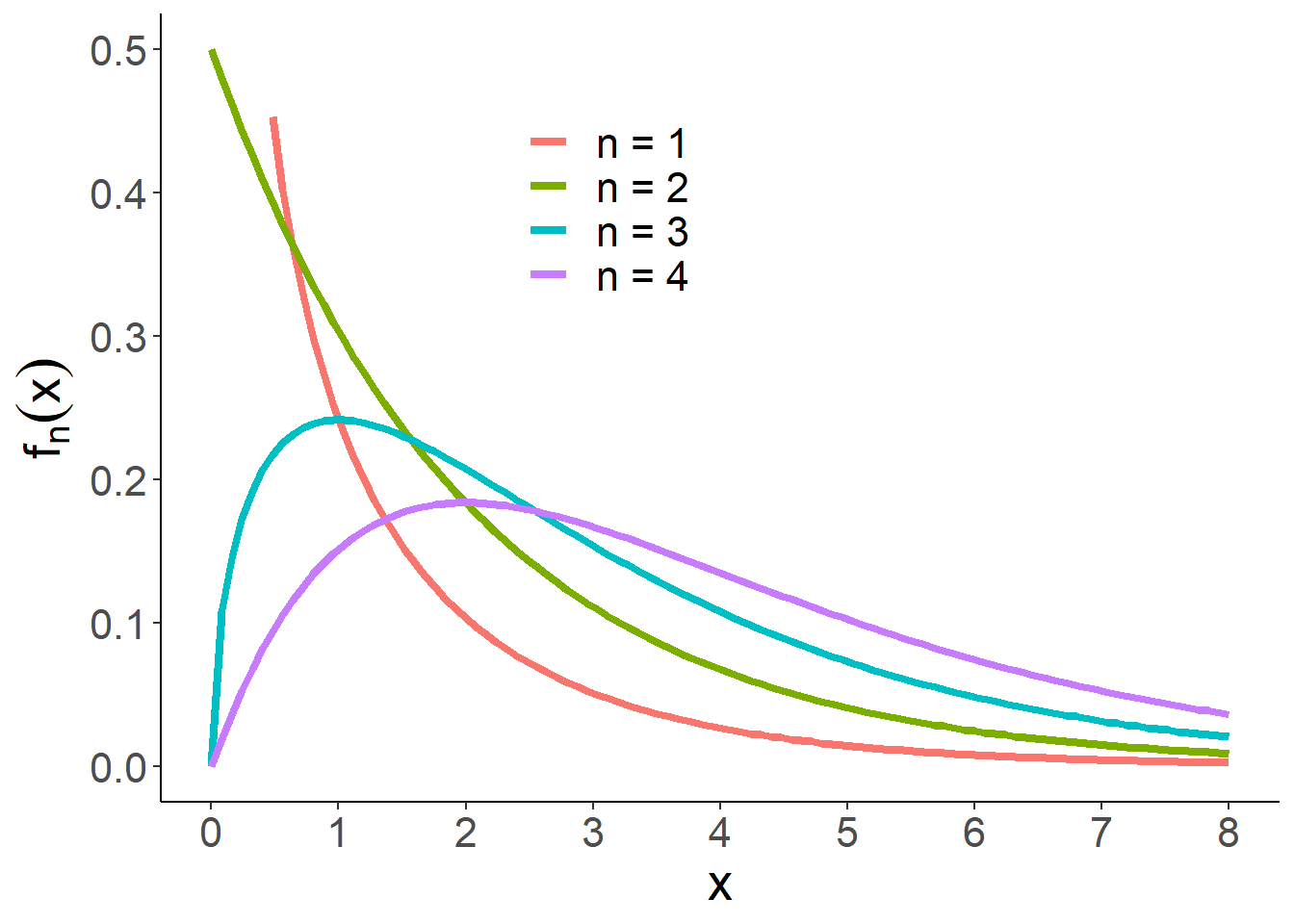### 1.6.3 正态总体抽样分布定理

• $$\bar{X}\sim N(\mu,\sigma^2/n)$$,
• $\frac{nS_n^2}{\sigma^2}=\frac{\sum_{i=1}^n(X_i-\bar{X})^2}{\sigma^2}\sim \chi^2(n-1),$
• 样本均值$$\bar{X}$$与样本方差$$S_n^2$$相互独立。

\begin{align*} F_Y(y) &= P(Y_{1:n}\le y) = P(AX_{1:n}\le y) \\&=\int_{Ax\le y}\frac 1{(2\pi)^{n/2}|\Sigma|^{1/2}}e^{-(1/2)(x-\mu)^\top \Sigma^{-1}(x-\mu)}d x \\&=\int_{z\le y}|A^{-1}|\frac 1{(2\pi)^{n/2}|\Sigma|^{1/2}}e^{-(1/2)(A^{-1}z-\mu)^\top \Sigma^{-1}(A^{-1}z-\mu)}d z \\&=\int_{z\le y}\frac 1{(2\pi)^{n/2}|A\Sigma A^\top|^{1/2}}e^{-(1/2)(z-A\mu)^\top (A\Sigma A^\top)^{-1}(z-A\mu)}d z. \end{align*}

$A=\left[ \begin{matrix} \frac 1{\sqrt{n}} & \frac 1{\sqrt{n}} & \frac 1{\sqrt{n}} & \cdots & \frac1{\sqrt{n}}\\ \frac 1{\sqrt{2\times 1}} & -\frac 1{\sqrt{2\times1}} & 0 & \cdots & 0\\ \frac 1{\sqrt{3\times 2}} & \frac 1{\sqrt{3\times2}} & -\frac 2{\sqrt{3\times2}} & \cdots & 0\\ \vdots & \vdots & \vdots & \vdots & \vdots \\ \frac 1{\sqrt{n\times (n-1)}} & \frac 1{\sqrt{n\times (n-1)}} & \frac {1}{\sqrt{n\times (n-1)}} & \cdots & -\frac {n-1}{\sqrt{n\times (n-1)}}\\ \end{matrix} \right].$

$$Y_{1:n}=AX_{1:n}$$. 由上面引理得，$$Y_{1:n}\sim N((\sqrt{n} \mu,0,\dots,0)^\top,\sigma^2 I_n)$$. 注意到，$$Y_1 = \sqrt{n}\bar X$$, 所以$$\bar X=Y_1/\sqrt{n}\sim N(\mu,\sigma^2/n)$$. 又$\sum_{i=1}^n Y_i^2 = Y_{1:n}^\top Y_{1:n} = (A X_{1:n})^\top A X_{1:n}=X_{1:n}^\top X_{1:n}=\sum_{i=1}^n X_i^2.$

### 1.6.4 t分布

$T = \frac{X}{\sqrt{Y/n}}$ 的分布为自由度为$$n$$学生氏t分布（简称t分布），记为$$T\sim t(n)$$。其密度函数为：

$f_n(x)= \frac{\Gamma\left(\frac{n+1}2\right)}{\sqrt{n\pi }\Gamma\left(\frac n2\right)}\left(1+\frac{x^2}n\right)^{-\frac{n+1}{2}}.$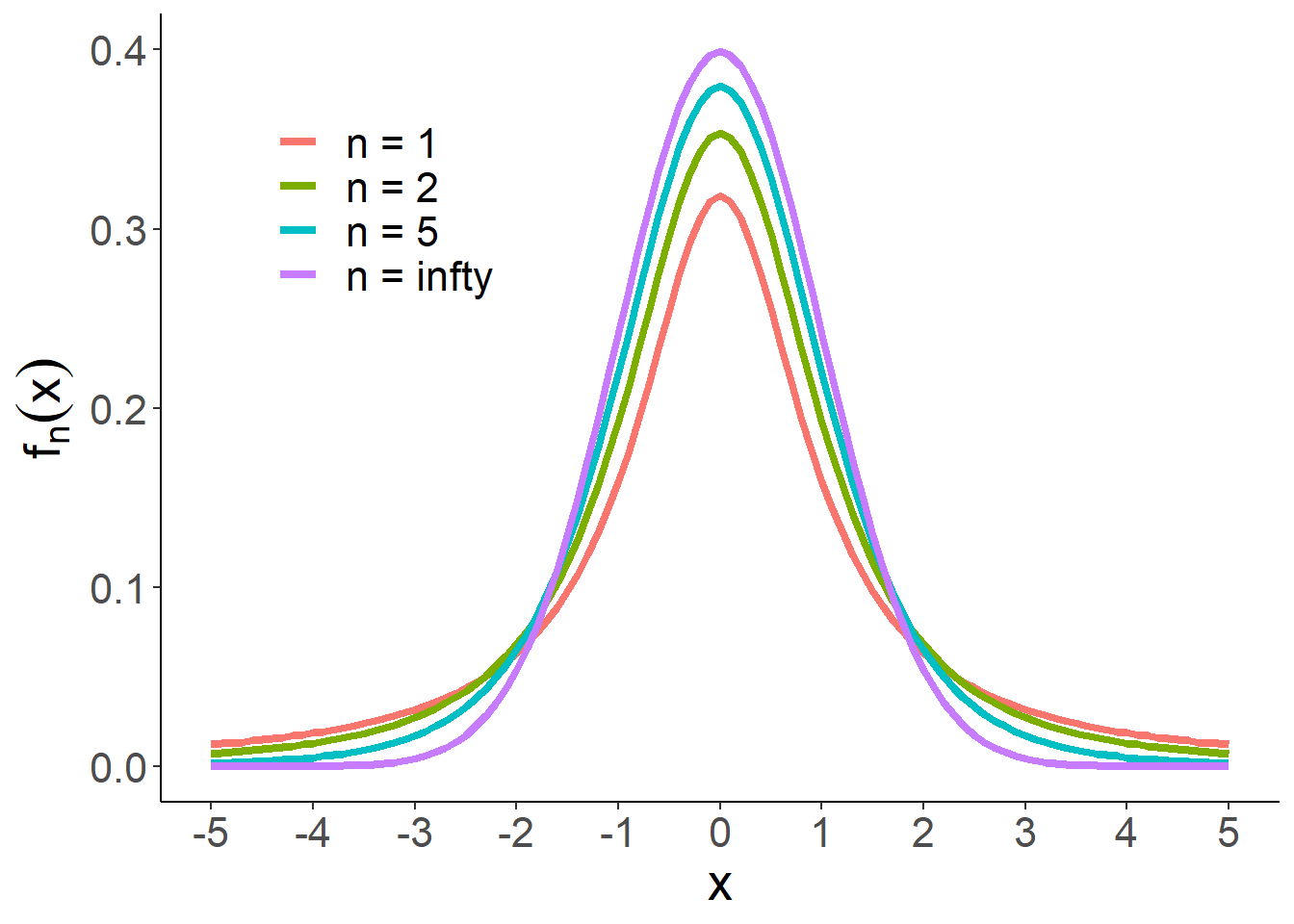t分布的两种特例：

• $$n=1$$时，t分布成为柯西分布。
• 可以证明：$$\lim_{n\to\infty}f(x)=\frac{1}{\sqrt{2\pi}}e^{-\frac{x^2}{2}}$$. 当$$n\ge 25$$时,可以认为t分布与$$N(0,1)$$接近。

t分布的起源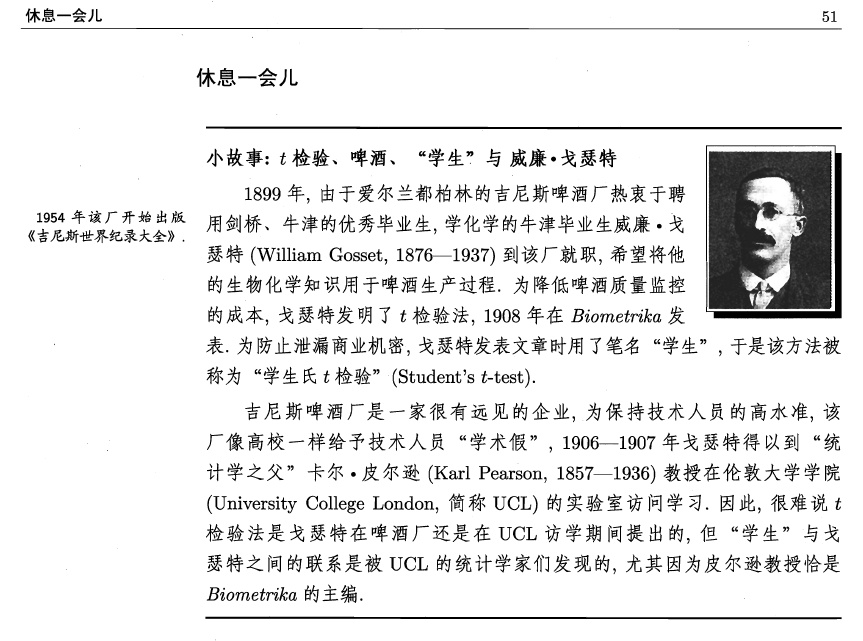### 1.6.5 样本均值与标准差之比的抽样分布

$\frac{\bar{X}-\mu}{S_n/\sqrt{n-1}}=\frac{\bar{X}-\mu}{S_n^*/\sqrt{n}}\sim t(n-1).$

$\frac{\bar{X}-\mu}{\sigma/\sqrt{n}}\sim N(0,1)$

$$X\sim N(0,1)$$ 0.0455 0.0124 0.0027 0.000465
$$X\sim t(4)$$ 0.1161 0.0668 0.0399 0.0249

### 1.6.6 F分布

$Z=\frac{X/m}{Y/n}$ 的分布为第一自由度为$$m$$、第二自由度为$$n$$的F分布，记$$Z\sim F(m,n)$$。其密度函数为

$f_{mn}(x)= \frac{\Gamma((m+n)/2)}{\Gamma(m/2)\Gamma(n/2)}\left(\frac{m}{n}\right)^{m/2}x^{\frac m2-1}(1+mx/n)^{-(m+n)/2} 1\{x>0\}.$

F分布的性质

• $$Z\sim F(m,n)$$, 则$$1/Z\sim F(n,m)$$.
• 如果$$T\sim t(n)$$, 则$$T^2\sim F(1,n)$$.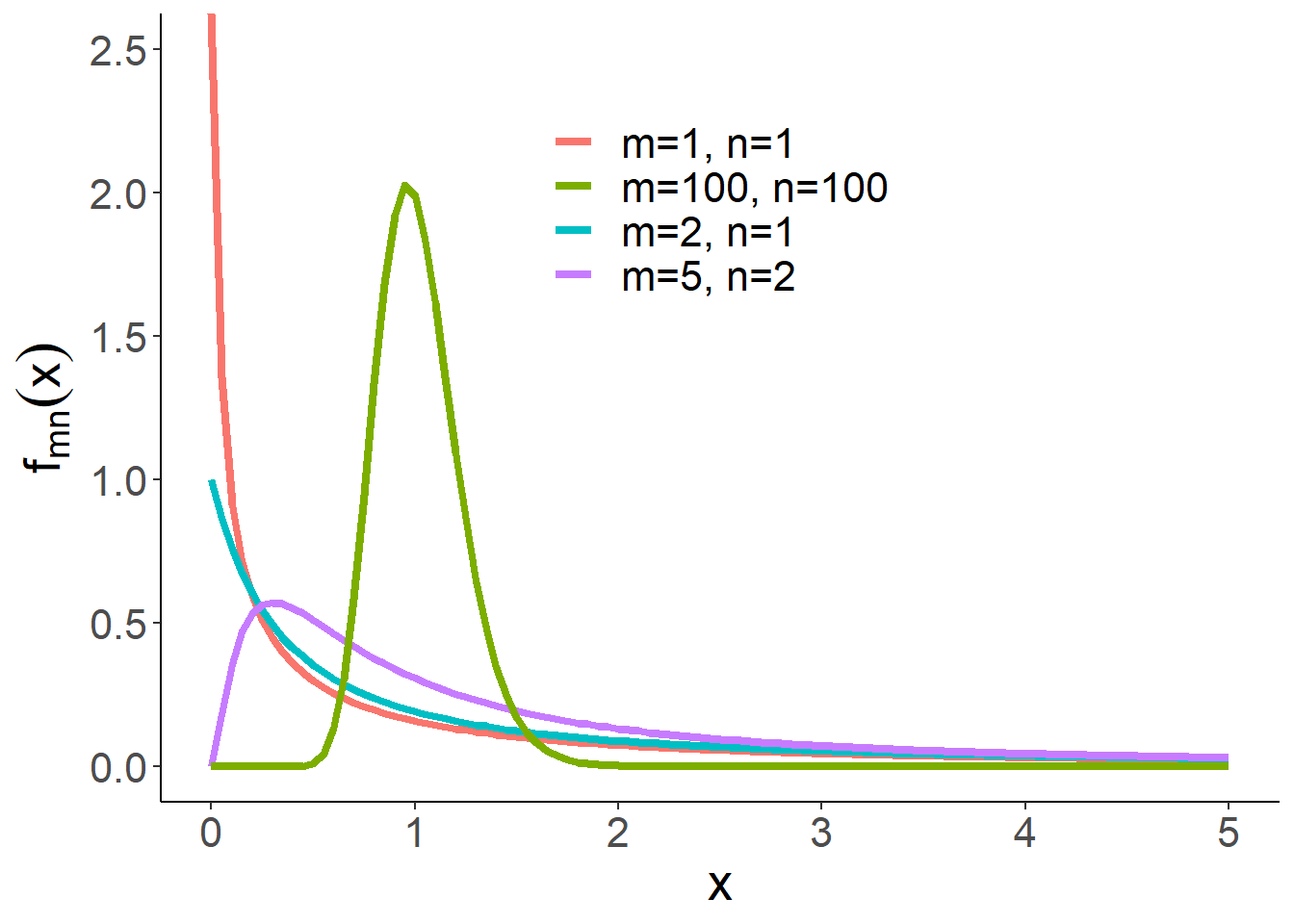### 1.6.7 两个独立正态总体的抽样分布

$\frac{(\bar X-\bar Y)-(\mu_1-\mu_2)}{\sqrt{\sigma_1^2/m+\sigma_2^2/n}}\sim N(0,1).$

$\frac{mS_{1m}^2/\sigma_1^2/(m-1)}{nS_{2n}^2/\sigma_2^2/(n-1)}=\frac{S_{1m}^{*2}\sigma_2^2}{S_{2n}^{*2}\sigma_1^2}\sim F(m-1,n-1).$

$\frac{(\bar X-\bar Y)-(\mu_1-\mu_2)}{S_w\sqrt{1/m+1/n}}\sim t(m+n-2),$

### 1.6.8 顺序统计量

$\begin{cases} F_{X_{(1)}}(x) = 1-(1-F(x))^n\\ f_{X_{(1)}}(x) = n(1-F(x))^{n-1}f(x). \end{cases}$ $$X_{(n)}=\max(X_1,\dots,X_n)$$的分布函数和密度函数分别

$\begin{cases} F_{X_{(n)}}(x) = F(x)^n\\ f_{X_{(n)}}(x) = nF(x)^{n-1}f(x). \end{cases}$ 更一般地，对任意$$k\in \{1,\dots,n\}$$

$f_{X_{(k)}}(x) = \frac{n!}{(n-k)!(k-1)!}F(x)^{k-1}[1-F(x)]^{n-k}f(x).$

\begin{align*} f_{X_{(i)},X_{(j)}}(x,y) &= \frac{n!}{(i-1)!(j-i-1)!(n-j)!}F(x)^{i-1} [F(y)-F(x)]^{j-i-1}\\ &\times [1-F(y)]^{n-j}f(x)f(y) 1\{x\le y\}. \end{align*}

$f(y_1,\dots,y_n)= \begin{cases} n!\prod_{i=1}^nf(y_i),&y_1<y_2<\dots<y_n\\ 0,&else. \end{cases}$

## 1.7 分位数

$x_\alpha=F^{-1}(\alpha)=\inf\{t\in\mathbb{R}|F(t)\ge \alpha\}.$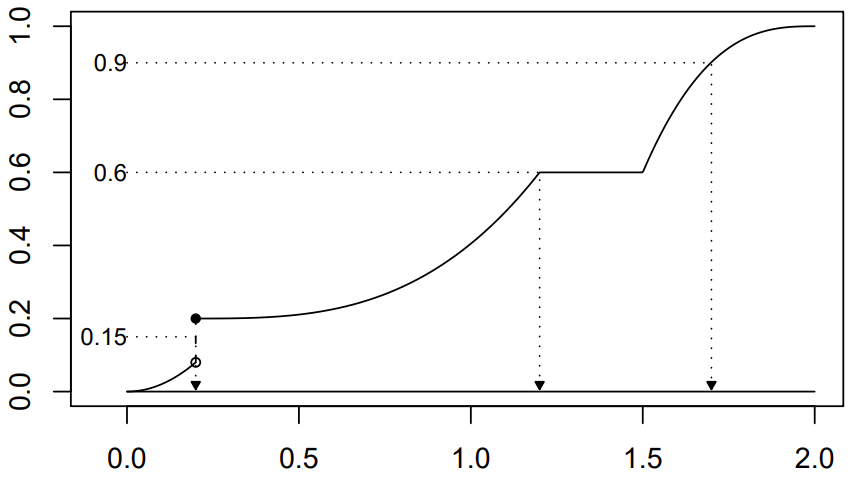• 标准正态分布分位数记为$$u_{\alpha}$$
• $$t$$分布分位数记为$$t_{\alpha}(n)$$
• $$\chi^2$$分布分位数记为$$\chi^2_{\alpha}(n)$$
• $$F$$分布分位数记为$$F_{\alpha}(m,n)$$

• 在分位点表中对于标准正态分布、$$t$$分布和F分布只能查到$$\alpha>1/2$$的分位数，需利用以下对称性间接查$$\alpha<1/2$$的分位数：

$u_\alpha=-u_{1-\alpha},\ t_\alpha(n)=-t_{1-\alpha}(n),\ F_{\alpha}(m,n)=\frac{1}{F_{1-\alpha}(n,m)}.$

• 对于$$t(n)$$分布，由于当$$n\to \infty$$时，其极限分布为$$N(0,1)$$, 所以自由度$$n$$比较大时，$$t_{\alpha}(n)\approx u_{\alpha}$$.

• $$X\sim \chi^2(n)$$分布，由于当$$n\to \infty$$时，$$(X-n)/\sqrt{2n}\stackrel{d}\to N(0,1)$$, 所以自由度$$n$$比较大时，$$\chi^2_{\alpha}(n)\approx u_{\alpha}\sqrt{2n}+n$$.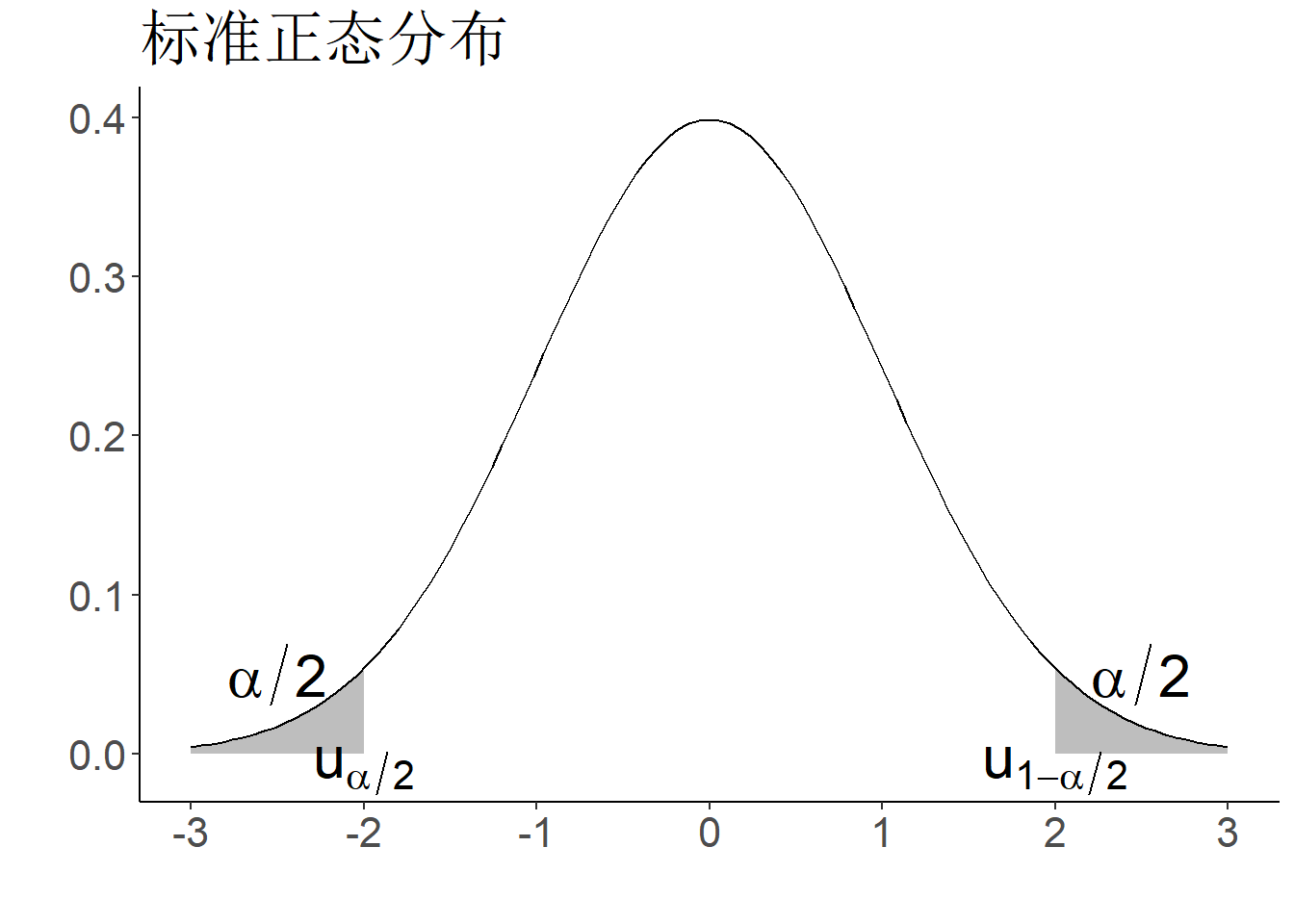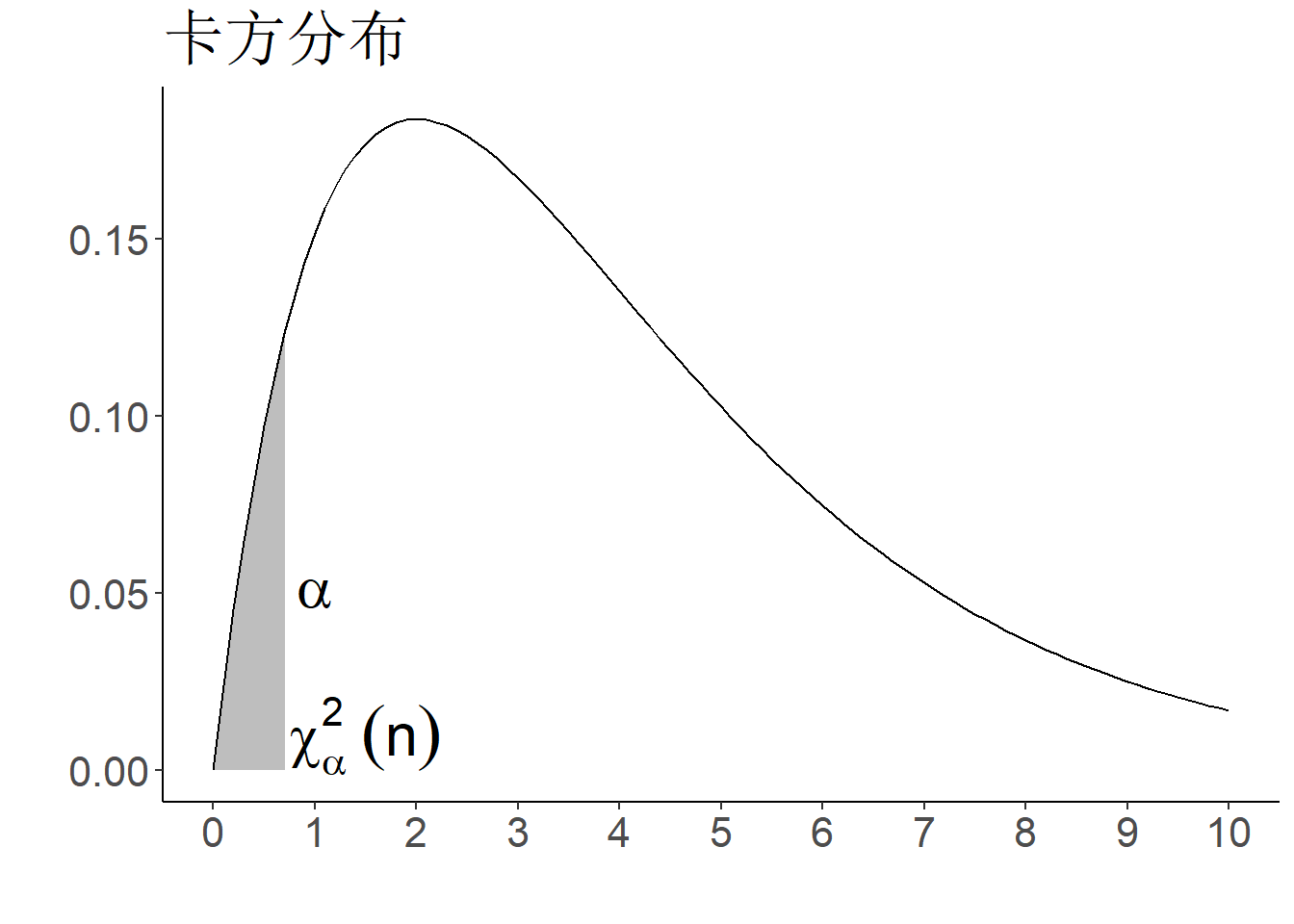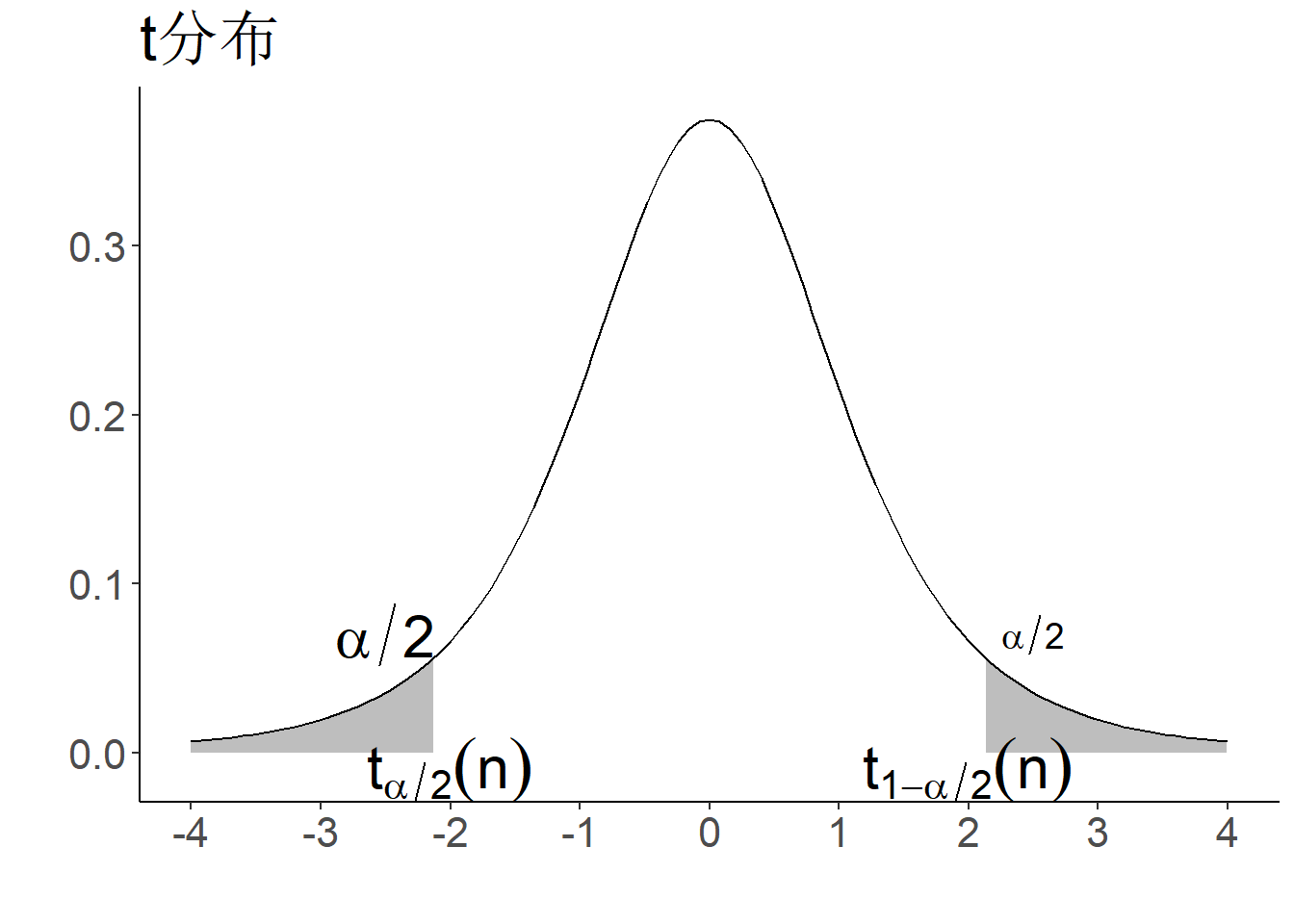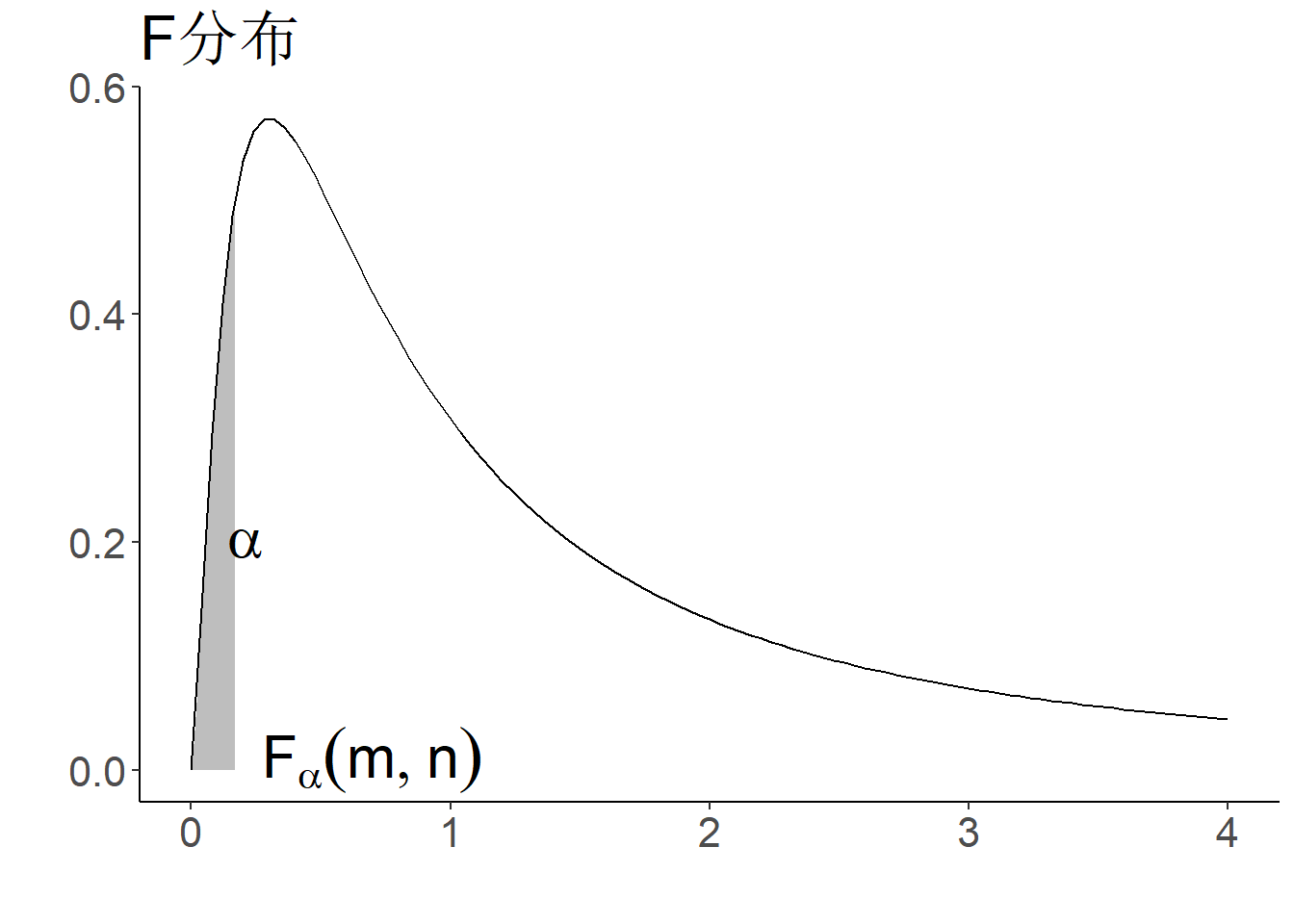## 1.8 本章习题

$p(x)=\frac k\lambda\left(\frac{x}{\lambda}\right)^{k-1}e^{-(x/\lambda)^k}1\{x\ge 0\},k>0,\lambda>0$

A. 是

B. 不是

A. $$T_1=x_1+x_2-2\mu$$

B. $$T_2=(x_1-\mu)/\sigma$$

C. $$T_3=(\bar x-10)/5$$

D. $$T_4=\frac 1 n\sum_{i=1}^n(x_i-S_n)^2$$

$n=15,\bar x_{n}=168, s_n=11.43, x_{n+1}=170.$

$$\bar x_{n+1},s_{n+1}^2$$，以及修正样本方差$$s_{n+1}^{*2}$$.

1. $$Y_1=X_1+X_2\sim Ga(\alpha_1+\alpha_2,\lambda)$$
2. $$Y_2=X_1/(X_1+X_2)\sim Beta(\alpha_1,\alpha_2)$$
3. $$Y_1$$$$Y_2$$独立

1. 求样本均值$$\bar X$$的分布；
2. $$\bar X$$落在区间$$(50.8,53.8)$$内的概率；
3. 若要以$$99\%$$的概率保证$$|\bar X-52|<2$$, 试问样本量至少应取多少？

A. $$u_{\alpha/2}$$

B. $$u_{1-\alpha/2}$$

C. $$u_{(1-\alpha)/2}$$

D. $$u_{1-\alpha}$$

A. $$\frac{\bar X-1}{2/\sqrt{n}}\sim t(n)$$

B. $$\frac{1}{4}\sum_{i=1}^n(X_i-1)^2\sim F(n,1)$$

C. $$\frac{\bar X-1}{\sqrt{2}/\sqrt{n}}\sim N(0,1)$$

D. $$\frac{1}{4}\sum_{i=1}^n(X_i-1)^2\sim \chi^2(n)$$

$Y=\frac{X_1^2+\dots+X_{10}^2}{2(X_{11}^2+\dots+X_{15}^2)}$

A. $$F(10,5)$$

B. $$F(11,4)$$

C. $$\chi^2(10)$$

D. 以上都不是

$p(x;\mu,\theta)=\frac 1\theta \exp\{-(x-\mu)/\theta\}, x>\mu,\theta>0$

$p_\theta(x)=\theta/x^2,\ 0<\theta<x<\infty$

$Y = (X_1+X_2)^2+(X_3+X_4)^2+(X_5+X_6)^2.$ If $$kY\sim \chi^2(3)$$, then $$k=$$?